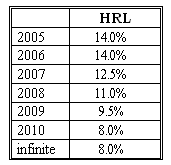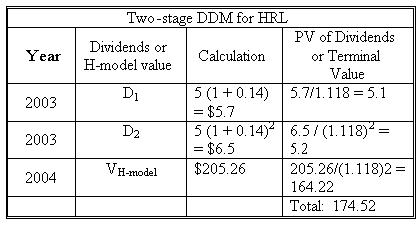### CFA Practice Question

There are 96 practice questions for this study session.

### CFA Practice Question

Beth is evaluating HRL Co. which started its business two years ago. Last year its growth was 14%. The risk-free rate is 4% and expected equity premium is 6%. Here is her forecast of the company's future dividend growth trend.After considerable study, she has decided to use the following information in her valuation (as of the end of 2004):

• Beta: 1.3.
• 2004 dividend: \$5.
• The risk-free rate: 4%.

What is the value of the HRL's stock?
Correct Answer: Company HRL can be valued using the three-stage DDM. Its growth is stable during the first two years. Then a period of linear transition is expected during 2007 - 2010 until the growth stabilizes at 8%. The required rate of return on HRL stock is rHRL = rf + beta x market premium = 0.04 + 1.3 x 0.06 = 0.118, or 11.8%.

We first calculate the value of HRL at the end of 2006 using the H-model with H= 2. VHRL2006 = [D2006 (1 + gL) + D2006 H(gS - gL)] / (r - gL) = [5 (1 + 0.14)2 (1 + 0.08) + 5 (1 + 0.14)2 x 2 x (0.14 - 0.08)] / (0.118 - 0.08) = \$205.26.

We discount this value along with the first two dividends to find the sock's present value:User Comment
danlan2 We can not use H-Model from 2004, but can use it from 2006, since the growth rate change is not constant.
Smiley225 agree, possibly a typo. But use H-model to 2006 value, then PV back to 2004. Then dont forget to PV the 2 dividends in 2005 and 2006.
rhardin I worked this out using the cashflow function in my calculator and got 316.70. Did any one else try to work it out manually?
REITboy Worked it out manually, and got \$170.34 based on the following CFs, beginning with C01: 5.70, 6.50, 7.31, 8.11, 8.89, and 9.60+272.63=282.23.
arudkov 2 EITboy - got almost the same (rounding influenced, CF1 5.675)
quanttrader use the H model from beg of yr 2007 to end of year 2010; ie H = 4 /2 =2. and then discount value back 2 years along with previous dividends.
quanttrader discount the H model terminal value by 2 periods (end of year 2006 to end of 2004), the 2006 dividend by 2 periods, and the 2005 dividend by 1 period.
philjoe I also got 170.34 doing it manually...
davidt876 you guys realise you're not supposed to be doing it 'normally' - right?

they explicitly ask you to calculate using the H-model on the exam, so you need to know how to use it. if you're not sure why the answers come out different, read the first 2 paragraphs of my answer on the previous question.